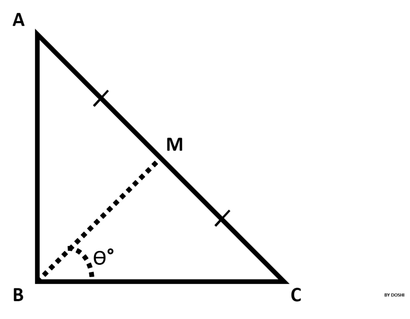## Algorithm

Problem Name: Python - Find Angle MBC

In this HackerRank Functions in PYTHON problem solution,ABC is a right triangle 90 degree at B.

Therefore <ABC = 90 degree

Point M is the midpoint of hypotenuse AC.

You are given the lengths AB and BC.

Input Format

The first line contains the length of side AB.

The second line contains the length of side BC.

Constraints

• 0 < A <= 100
• 0 < B <= 100
• Lengths AB and BC are natural numbers.

d

## Code Examples

### #1 Code Example with Python Programming

Code - Python Programming


import math

a = int(input())
b = int(input())

print(f'{math.degrees(math.atan(a / b)):1.0f}\xb0')

Copy The Code &